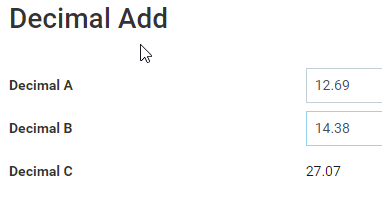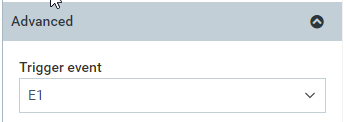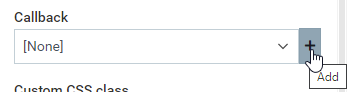# Displaying calculated amounts as default

Hi,

I need to create a field that displays an amount calculated from previous populated fields.

For example:

If field A = 1 and
field B = 1 then
field C (displays a sum of A and B) = 2

The calculations are more than just a sum, but this is just as an example.

I hope I’m making sense. Thank you

You need a Composite.

Example:

I’ve added “Field A” and “Field B” as Integer based properties in my User object, therefore to create the “Field C” composite I do the following:

Now for each record in the User object I will have a composite named “Field C” which will always have a value equal to “Field A” + “Field B”.

I hope this makes sense.

Morning Kalpana,

If you however wanted to do this inline at the point of entry within a form, you can do this using a callback function and events.In my example I have created an object with three values, Decimal A, Decimal B and Decimal C.
Decimal C will have a callback that will cause the value to be the sum of Decimal A and B.

Within page builder I add these properties to my form and for A and B in settings under advanced I ask them to trigger event E1.For Decimal C, which will display the answer, I ask it to Refresh on Event E1 so changing either A or B will cause C to recalculate. I then go to Callback and and click the add button.Within your fragment callback you need to first get the values of the field within the widget group using fragment_presenter.get_values();

To access the values and perform our sum we will need to create references to the property id’s so that we can reference them in the values array.

After this we can then pull our values and add them together.

values[cs.ref(“decimal_a”) retrieves the values for Decimal A.

We wrap this in parseFloat so that we can perform addition on a decimal not on a string. Without it we would append the two values rather than add them.

We use fragment_presenter.set_value() to set the value and finish the callback.

The code used:

main: function(fragment_presenter) {

``````    values = fragment_presenter.get_values();

fragment_presenter.set_value((parseFloat(values[cs.ref("decimal_a")]) + parseFloat(values[cs.ref("decimal_b")])) || '');

}
``````

The || ‘’, just sets the value to empty if the sum does not resolve to a valid answer.

Hope this helps.

@adam.mills Can this be achieved using a choice type and variable field? I’ve referenced them both in the same way as shown above but the calculation doesn’t work.

Afternoon Ross,
Can you explain more please when you say the calculation doesn’t work.

At what point does it fail?

Log out the result of your call back to detective at each stage, logging the fact the Action is being run and then all constituent values i.e. get_values and the desired set value. At what point is the error?